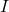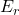## In a city with an air-pollution problem, a bus has no combustion engine. It runs on energy drawn from a large, rapidly rotating flywheel und

Question

In a city with an air-pollution problem, a bus has no combustion engine. It runs on energy drawn from a large, rapidly rotating flywheel under the floor of the bus. The flywheel is spun up to its maximum rotation rate of 4000 rev/min by an electric motor at the bus terminal. Every time the bus speeds up, the flywheel slows down slightly. The bus is equipped with regenerative braking so that the flywheel can speed up when the bus slows down. The flywheel is a uniform solid cylinder with mass 1600 kg and radius 0.650 m. The bus body does work against air resistance and rolling resistance at the average rate of 18.0 hp as it travels with an average speed of 40 km/h. How far can the bus travel before the flywheel has to be spun up to speed again

in progress 0
2 months 2021-08-21T23:26:31+00:00 1 Answers 2 views 0

the bus can travel 24.54 km before the flywheel has to be spun up to speed again

Explanation:

Given the data in the question;

mass of wheel m = 1600 kg

radius of the fly wheel r = 0.650 m

angular speed of the wheel ω = 4000 rev/min = 418.879 rad/s

Average speed of the bus v = 40 km/h =  11.1111 m/s

Power p = 18 hp = 13428 watt

So moment of inertia of the fly wheel is;=mr²

we substitute=× 1600 kg × (0.650 m)²= 338 kgm²

So, the rotational energy of the bus will be;=$$I$$ω²

we substitute=× 338 kgm²  × ( 418.879 rad/s )= 29652675.212329 J

relation of power to energy

P = E / t

but we know that time t = distance / velocity

t = d/v

P = E / ( d/v)

we solve for distance (d)

P = Ev / d

Pd = Ev

d = Ev / P

so we substitute

d = [( 29652675.212329 J )( 11.1111 m/s )] / [ 13428 W ]

d = 24536.33 m

d = 24.54 km

Therefore, the bus can travel 24.54 km before the flywheel has to be spun up to speed again